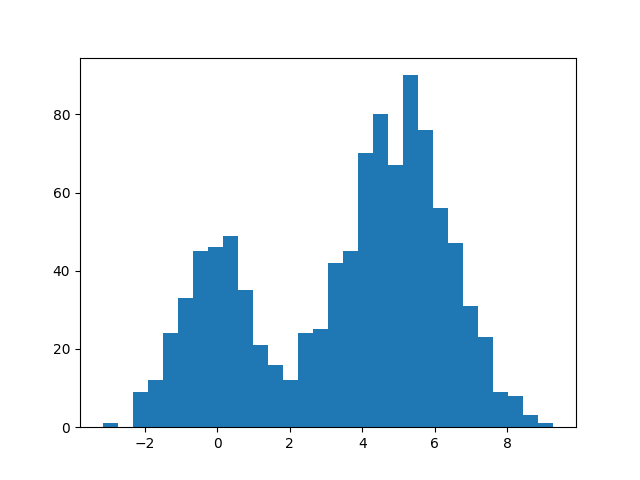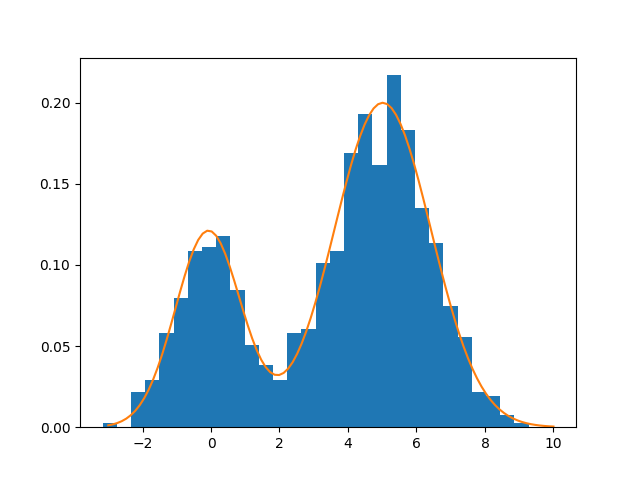（先啰嗦一句：本文的标题和内容牵涉到 TensorFlow，只是因为它是可用的工具之一，我相信很多其他的框架都可以做到文中我想要实现的功能。我自己并没有工具上的偏好，所以就当是拿 TensorFlow 举一个例子。）

import numpy as np
import matplotlib.pyplot as plt

np.random.seed(123)

# Parameters
p1 = 0.3
p2 = 0.7
mu1 = 0.0
mu2 = 5.0
sigma1 = 1.0
sigma2 = 1.5

# Simulate data
N = 1000
x = np.zeros(N)
ind = np.random.binomial(1, p1, N).astype('bool_')
n1 = ind.sum()
x[ind] = np.random.normal(mu1, sigma1, n1)
x[np.logical_not(ind)] = np.random.normal(mu2, sigma2, N - n1)

# Histogram
plt.hist(x, bins=30)
plt.show()import tensorflow as tf
import tensorflow.contrib.distributions as ds

# Define data
t_x = tf.placeholder(tf.float32)

# Define parameters
t_p1_ = tf.Variable(0.0, dtype=tf.float32)
t_p1 = tf.nn.softplus(t_p1_)
t_mu1 = tf.Variable(0.0, dtype=tf.float32)
t_mu2 = tf.Variable(1.0, dtype=tf.float32)
t_sigma1_ = tf.Variable(1.0, dtype=tf.float32)
t_sigma1 = tf.nn.softplus(t_sigma1_)
t_sigma2_ = tf.Variable(1.0, dtype=tf.float32)
t_sigma2 = tf.nn.softplus(t_sigma2_)

# Define model and objective function
t_gm = ds.Mixture(
cat=ds.Categorical(probs=[t_p1, 1.0 - t_p1]),
components=[
ds.Normal(t_mu1, t_sigma1),
ds.Normal(t_mu2, t_sigma2)
]
)
t_ll = tf.reduce_mean(t_gm.log_prob(t_x))

# Optimization
train = optimizer.minimize(-t_ll)

# Run
sess = tf.Session()
init = tf.global_variables_initializer()
sess.run(init)
for _ in range(500):
sess.run(train, {t_x: x})

print('Estimated values:', sess.run([t_p1, t_mu1, t_mu2, t_sigma1, t_sigma2]))
print('True values:', [p1, mu1, mu2, sigma1, sigma2])


Estimated values: [0.29020751, -0.078560337, 5.0137568, 0.95754921, 1.4157518]
True values: [0.3, 0.0, 5.0, 1.0, 1.5]